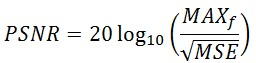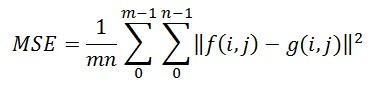Peak Signal-to-Noise Ratio as an Image Quality Metric

Overview

This article describes the Peak Signal-to-Noise ratio as applied to images as a quality metric. The article describes developing the metrics in LabVIEW and suggests how they can be used. The functions are included as sample downloads.

Introduction

The term peak signal-to-noise ratio (PSNR) is an expression for the ratio between the maximum possible value (power) of a signal and the power of distorting noise that affects the quality of its representation.  Because many signals have a very wide dynamic range, (ratio between the largest and smallest possible values of a changeable quantity) the PSNR is usually expressed in terms of the logarithmic decibel scale.

Image enhancement or improving the visual quality of a digital image can be subjective.  Saying that one method provides a better quality image could vary from person to person.   For this reason, it is necessary to establish quantitative/empirical measures to compare the effects of image enhancement algorithms on image quality.

Using the same set of tests images, different image enhancement algorithms can be compared systematically to identify whether a particular algorithm produces better results.  The metric under investigation is the peak-signal-to-noise ratio.  If we can show that an algorithm or set of algorithms can enhance a degraded known image to more closely resemble the original, then we can more accurately conclude that it is a better algorithm.

Mathematics

For the following implementation, let us assume we are dealing with a standard 2D array of data or matrix.  The dimensions of the correct image matrix and the dimensions of the degraded image matrix must be identical.
The mathematical representation of the PSNR is as follows:Figure 1 - Peak Signal-to-Noise Equation

where the MSE (Mean Squared Error) is:Figure 2 - Mean Squared Error Equation

This can also be represented in a text based format as:

MSE = (1/(m*n))*sum(sum((f-g).^2))

PSNR = 20*log(max(max(f)))/((MSE)^0.5)

Legend:
f
represents the matrix data of our original image
g represents the matrix data of our degraded image in question
m represents the numbers of rows of pixels of the images and i represents the index of that row
n represents the number of columns of pixels of the image and j represents the index of that column
MAXf is the maximum signal value that exists in our original “known to be good” image

Motivation for Use as an Image Quality Metric

The mean squared error (MSE) for our practical purposes allows us to compare the “true” pixel values of our original image to our degraded image.   The MSE represents the average of the squares of the "errors" between our actual image and our noisy image. The error is the amount by which the values of the original image differ from the degraded image.

The proposal is that the higher the PSNR, the better degraded image has been reconstructed to match the original image and the better the reconstructive algorithm.  This would occur because we wish to minimize the MSE between images with respect the maximum signal value of the image.

Closing Notes

When you try to compute the MSE between two identical images, the value will be zero and hence the PSNR will be undefined (division by zero).  The main limitation of this metric is that it relies strictly on numeric comparison and does not actually take into account any level of biological factors of the human vision system such as the structural similarity index. (SSIM)

For colour images, the MSE is taken over all pixels values of each individual channel and is averaged with the number of colour channels.  Another option may be to simply perform the PSNR over a converted luminance or grayscale channel as the eye is generally four times more susceptible to luminance changes as opposed to changes in chrominance.  This approximation is left up to the experimenter.